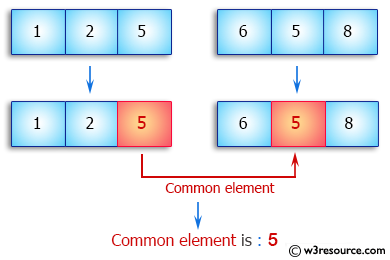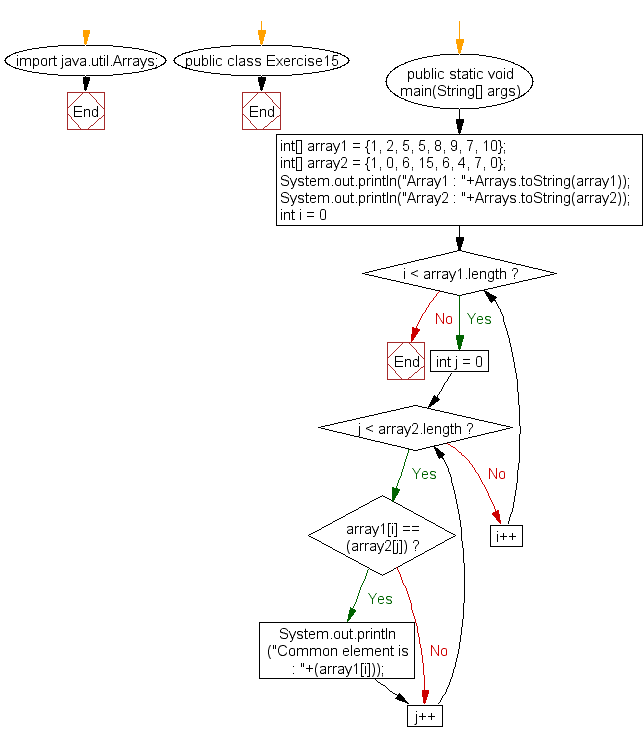﻿ Java: Find the common elements between two arrays# Java Array Exercises: Find the common elements between two arrays of integers

## Java Array: Exercise-15 with Solution

Write a Java program to find the common elements between two arrays of integers.

Pictorial Presentation:Sample Solution:

Java Code:

``````import java.util.Arrays;
public class Exercise15 {
public static void main(String[] args)
{
int[] array1 = {1, 2, 5, 5, 8, 9, 7, 10};
int[] array2 = {1, 0, 6, 15, 6, 4, 7, 0};

System.out.println("Array1 : "+Arrays.toString(array1));
System.out.println("Array2 : "+Arrays.toString(array2));

for (int i = 0; i < array1.length; i++)
{
for (int j = 0; j < array2.length; j++)
{
if(array1[i] == (array2[j]))
{

System.out.println("Common element is : "+(array1[i]));
}
}
}

}
}
```
```

Sample Output:

```Array1 : [1, 2, 5, 5, 8, 9, 7, 10]
Array2 : [1, 0, 6, 15, 6, 4, 7, 0]
Common element is : 1
Common element is : 7
```

Flowchart:Visualize Java code execution (Python Tutor):

Java Code Editor:

Improve this sample solution and post your code through Disqus

What is the difficulty level of this exercise?

Test your Programming skills with w3resource's quiz.

﻿

## Java: Tips of the Day

countOccurrences

Counts the occurrences of a value in an array.

Use Arrays.stream().filter().count() to count total number of values that equals the specified value.

```public static long countOccurrences(int[] numbers, int value) {
return Arrays.stream(numbers)
.filter(number -> number == value)
.count();
}
```

Ref: https://bit.ly/3kCAgLb